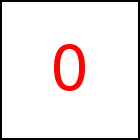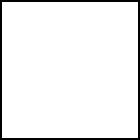# from Library Method

Short Description: Set Start Point of Motion

Signature: t.from (x0,y0,z0) or (p0)
Group: Motion
Class: transition Class

Sets the starting position of the motion of the animated object. (x0,y0,z0) are Coordinates. Alternatively (p0) is a Position Specification that will be resolved into coordinates. Default of the starting position is the position of the animated HTML element.

from may be used once on a single transition unless the transition was created using certain forms of cont that do from implicitely.

### Examples

 taccgl.actor("testimg").from(1000,2000,0).start(); RUN taccgl.actor("testimg").from(1000,2000,0).to(0,400,0).start(); RUN taccgl.actor("testimg").from(0,0,1000).start(); RUN taccgl.actor("testimg").from(0,0,-1000).start(); RUN taccgl.actor("pgMiddleColumnTable").from(0,0,-30000).start(); RUN taccgl.actor("pgMiddleColumnTable").from(0,0,-30000).start(); taccgl.actor("testimg").start();taccgl.actor("LeftcolumnDown").start(); RUN var a=taccgl.actor("testimg"); a.from(a.x0,a.y0+500,0).start(); RUN var a=taccgl.actor("pgMiddleColumnTable"); a.from(a.x0,0,0).start(); RUN

WebGL™ is a trademark of the Khronos Group Inc.

Next Page:transition.position - Set Position of Object
Previous Page: transition.to - Set End Point of Motion

# Motion

taccgl™ - Canvas Library